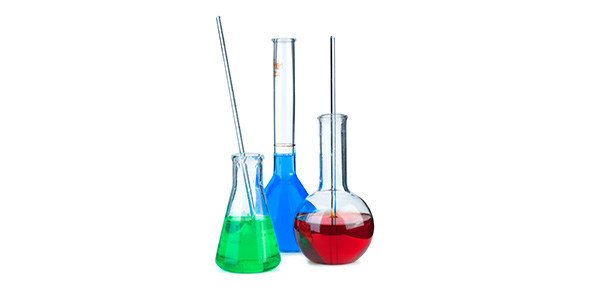# Chemical Calculations Notes 1

10 Questions | Attempts: 242
ShareSettings• 1.
Example 1B How many carbon atoms are present in 1.00mol of ethane, C2H6?
• 2.
Example 1c How many moles of O2 is present in 4.00 x 1022 molecules of oxygen gas?
• 3.
Example 1d How many moles of oxygen atoms are present in 2.00 x 1023 molecules of oxygen gas?
• 4.
Example 3c How many oxygen atoms have the same mass as one bromine atom?
• 5.
What is the relative molecular mass of dinitrogen pentoxide N2O5?
• 6.
What is the relative molecular mass of oxygen? Remember the oxygen here we are talking about is oxygen gas O2.
• 7.
Example 5b What is the relative formula mass of lithium oxide, Li2O?
• 8.
Example 5c What is the relative formula mass of copper sulphate pentahydrate CuSO4.5H2O?
• 9.
Example 6b What is the mass of 0.500mol of water?
• 10.
Example 6c How many mole of oxygen is present in a bag filled with 64.0g of oxygen gas?

## Related TopicsBack to top
×

Wait!
Here's an interesting quiz for you.